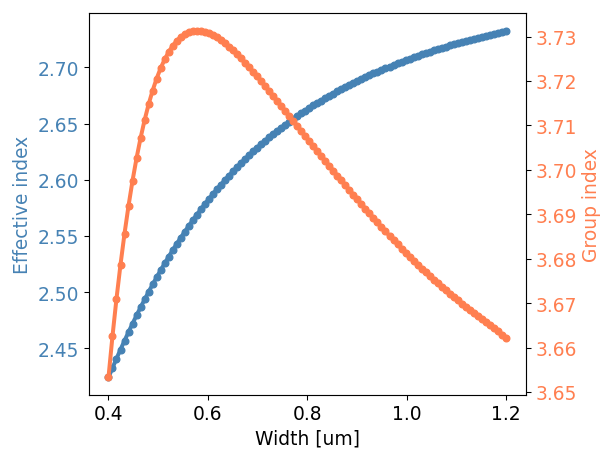# Waveguides¶

SiFab contains two types of waveguide templates:

## Wire waveguide templates¶

SiFab contains silicon wire waveguide templates, which have a rectangular cross-section fully etched through the silicon layer.

• The waveguide template SiWireWaveguideTemplate is parametric in width and wavelength. It contains all the cross-sectional information about the wire waveguide: how to draw the layout and the simulation model parameters, such as the effective and group indices.
• Several fixed-width templates are defined: SWG450, SWG550, SWG1000.

Example

Based on the waveguide template, we can define a waveguide following a given shape, plot its layout and visualize its virtual fabrication (top-down and cross-section). We can also plot the power and phase transmission as a function of wavelength.

""" Plot layout, cross-section and transmission of a silicon wire waveguide.
"""

import si_fab.all as pdk
import ipkiss3.all as i3
import numpy as np
import pylab as plt

center_env = i3.Environment(wavelength=1.55)

# Waveguide template: it contains all the cross-sectional information.
wg_tmpl = pdk.SiWireWaveguideTemplate()
wg_tmpl.Layout(core_width=0.47)
wg_tmpl_cm = wg_tmpl.CircuitModel()

print("neff @ 1.55: {}".format(wg_tmpl_cm.get_n_eff(center_env)))
print("ng @ 1.55: {}".format(wg_tmpl_cm.get_n_g(center_env)))
print("loss @ 1.55: {}".format(wg_tmpl_cm.get_loss_dB_m(center_env)))

# Waveguide layout and virtual fabrication
wg = i3.RoundedWaveguide(trace_template=wg_tmpl)
wg_lo = wg.Layout(shape=[(0.0, 0.0), (20.0, 0.0), (40.0, 20.0), (60.0, 20.0)])
wg_lo.visualize(annotate=True)
wg_lo.visualize_2d(process_flow=i3.TECH.VFABRICATION.PROCESS_FLOW_FEOL)
xs = wg_lo.cross_section(cross_section_path=i3.Shape([(1.0, 2.0), (1.0, -2.0)]))
xs.visualize()

# Plot the power and phase transmission as a function of wavelength
wavelengths = np.linspace(1.545, 1.555, 10)
wg_cm = wg.CircuitModel()
wg_S = wg_cm.get_smatrix(wavelengths)
transmission = wg_S["out", "in"]

fig, (ax1, ax2) = plt.subplots(2, 1, sharex=True)

ax1.plot(wavelengths, np.abs(transmission)**2, 'bo-', markersize=5, linewidth=3)
ax1.ticklabel_format(axis='y', style='sci', scilimits=(-2, 2))
ax1.set_ylim(0.4 * 1e-6 + 9.99686e-1, 3.0 * 1e-6 + 9.99686e-1)
ax1.set_ylabel('Power transmission [dB]')

ax2.plot(wavelengths, np.unwrap(np.angle(transmission)), 'bo-', markersize=5, linewidth=3)
ax2.set_ylim(-10, 0)
ax2.set_xlabel('Wavelength [um]')
ax2.set_ylabel('Phase')

plt.show()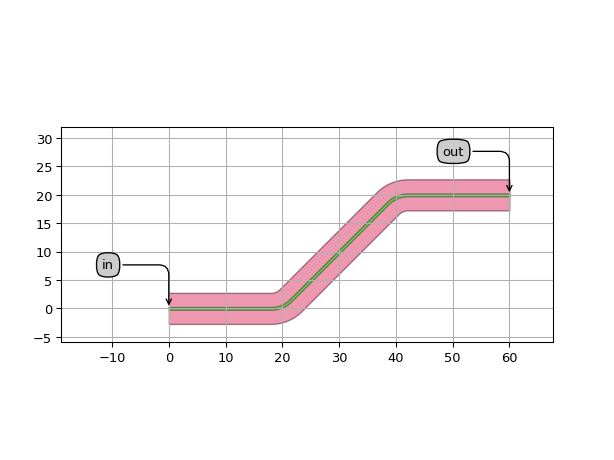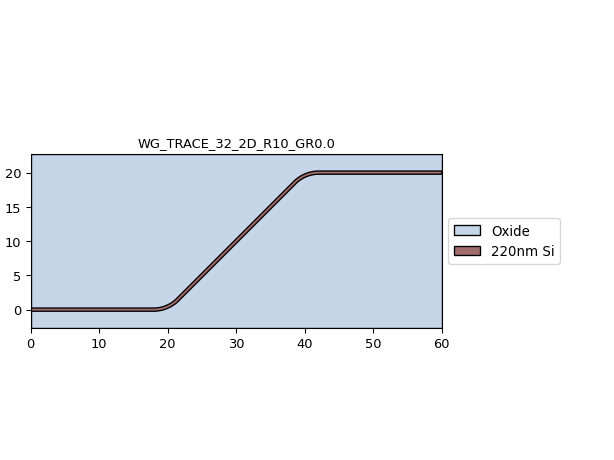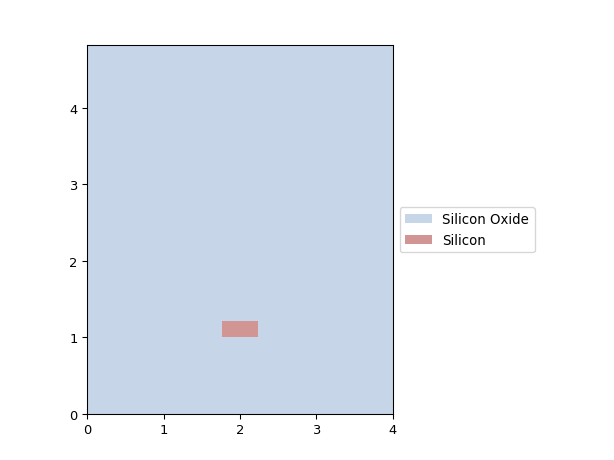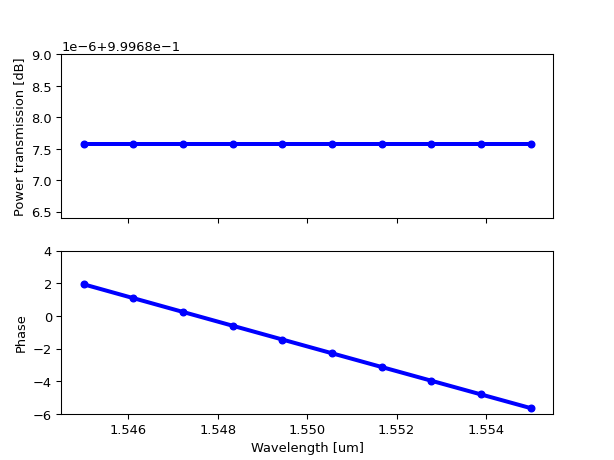### Dispersion diagram¶

The effective and group indices of the waveguide as a function of width can be visualized as follows:

"""Plot waveguide template dispersion diagram: neff/ng as function of width.
"""

import si_fab.all as pdk
import ipkiss3.all as i3
import numpy as np
import pylab as plt

center_env = i3.Environment(wavelength=1.55)
widths = np.linspace(0.4, 1.2, 100)
neffs = []
ngs = []

for w in widths:
tmpl = pdk.SiWireWaveguideTemplate()
tmpl.Layout(core_width=w)
tmpl_cm = tmpl.CircuitModel()
neffs.append(tmpl_cm.get_n_eff(center_env))
ngs.append(tmpl_cm.get_n_g(center_env))

fig, ax1 = plt.subplots()

ax1.plot(widths, neffs, 'bo-', markersize=5, linewidth=3, color='steelblue')
ax1.set_xlabel('Width [um]', fontsize=14)
ax1.set_ylabel('Effective index', color='steelblue', fontsize=14)
ax1.tick_params(axis='y', labelcolor='steelblue', labelsize=14)
ax1.tick_params(axis='x', labelcolor='black', labelsize=14)

ax2 = ax1.twinx()
ax2.plot(widths, ngs, 'ro-', markersize=5, linewidth=3, color='coral')
ax2.set_ylabel('Group index', color='coral', fontsize=14)
ax2.tick_params(axis='y', labelcolor='coral', labelsize=14)

fig.tight_layout()
plt.show()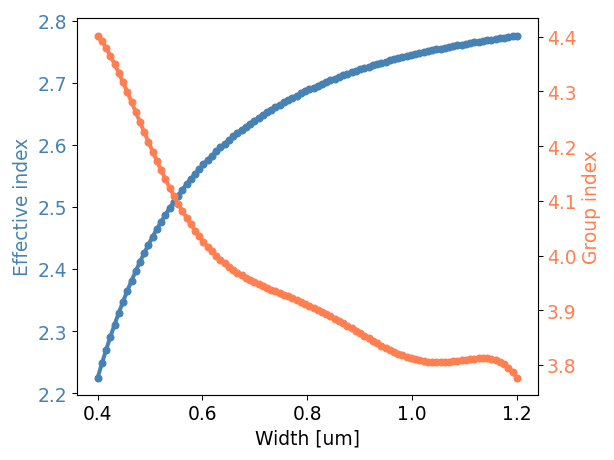## Rib waveguide templates¶

SiFab contains silicon rib waveguide templates, which have a rectangular cross-section shallow etched through the silicon layer.

• The waveguide template SiRibWaveguideTemplate is parametric in width and wavelength. It contains all the cross-sectional information about the wire waveguide: how to draw the layout and the simulation model parameters, such as the effective and group indices.
• Several fixed-width templates are defined: RWG450, RWG850.

Example

Based on the waveguide template, we can define a waveguide following a given shape, plot its layout and visualize its virtual fabrication (top-down and cross-section). We can also plot the power and phase transmission as a function of wavelength.

""" Plot layout, cross-section and transmission of a silicon rib waveguide.
"""

import si_fab.all as pdk
import ipkiss3.all as i3
import numpy as np
import pylab as plt

center_env = i3.Environment(wavelength=1.55)

# Waveguide template: it contains all the cross-sectional information.
wg_tmpl = pdk.SiRibWaveguideTemplate()
wg_tmpl.Layout(core_width=0.47)
wg_tmpl_cm = wg_tmpl.CircuitModel()

print("neff @ 1.55: {}".format(wg_tmpl_cm.get_n_eff(center_env)))
print("ng @ 1.55: {}".format(wg_tmpl_cm.get_n_g(center_env)))
print("loss @ 1.55: {}".format(wg_tmpl_cm.get_loss_dB_m(center_env)))

# Waveguide layout and virtual fabrication
wg = i3.RoundedWaveguide(trace_template=wg_tmpl)
wg_lo = wg.Layout(shape=[(0.0, 0.0), (20.0, 0.0), (40.0, 20.0), (60.0, 20.0)])
wg_lo.visualize(annotate=True)
wg_lo.visualize_2d(process_flow=i3.TECH.VFABRICATION.PROCESS_FLOW_FEOL)
xs = wg_lo.cross_section(cross_section_path=i3.Shape([(1.0, 2.0), (1.0, -2.0)]))
xs.visualize()

# Plot the power and phase transmission as a function of wavelength
wavelengths = np.linspace(1.545, 1.555, 10)
wg_cm = wg.CircuitModel()
wg_S = wg_cm.get_smatrix(wavelengths)
transmission = wg_S["out", "in"]

fig, (ax1, ax2) = plt.subplots(2, 1, sharex=True)

ax1.plot(wavelengths, np.abs(transmission)**2, 'bo-', markersize=5, linewidth=3)
ax1.ticklabel_format(axis='y', style='sci', scilimits=(-2, 2))
ax1.set_ylim(0.2 * 1e-5 + 9.9843e-1, 1.6 * 1e-5 + 9.9843e-1)
ax1.set_ylabel('Power transmission [dB]')

ax2.plot(wavelengths, np.unwrap(np.angle(transmission)), 'bo-', markersize=5, linewidth=3)
ax2.set_ylim(-10, 0)
ax2.set_xlabel('Wavelength [um]')
ax2.set_ylabel('Phase')

plt.show()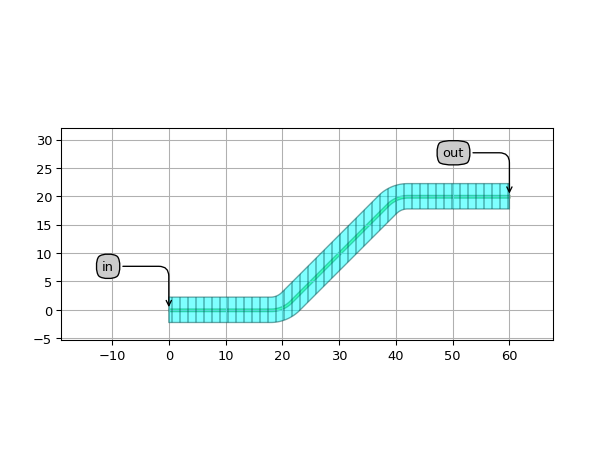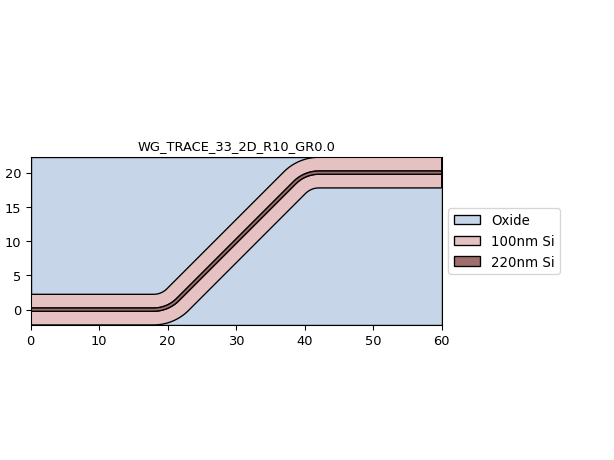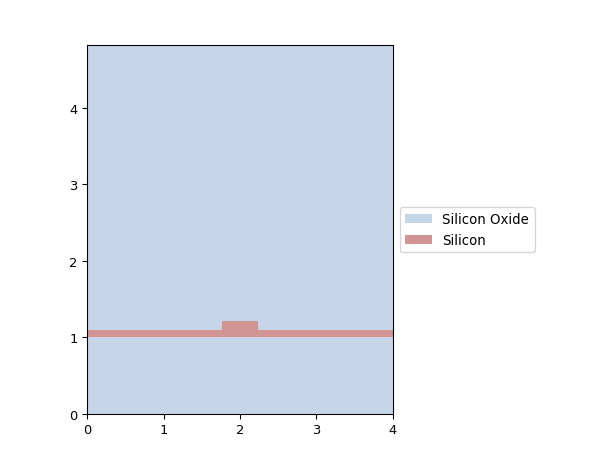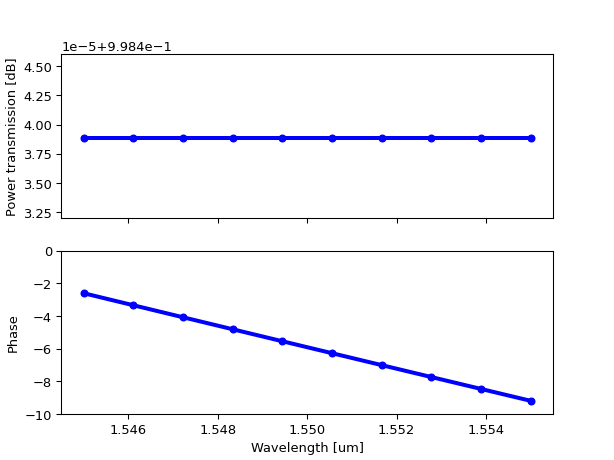### Dispersion diagram¶

The effective and group indices of the waveguide as a function of width can be visualized as follows:

"""Plot waveguide template dispersion diagram: neff/ng as function of width.
"""

import si_fab.all as pdk
import ipkiss3.all as i3
import numpy as np
import pylab as plt

center_env = i3.Environment(wavelength=1.55)
widths = np.linspace(0.4, 1.2, 100)
neffs = []
ngs = []

for w in widths:
tmpl = pdk.SiRibWaveguideTemplate()
tmpl.Layout(core_width=w)
tmpl_cm = tmpl.CircuitModel()
neffs.append(tmpl_cm.get_n_eff(center_env))
ngs.append(tmpl_cm.get_n_g(center_env))

fig, ax1 = plt.subplots()

ax1.plot(widths, neffs, 'bo-', markersize=5, linewidth=3, color='steelblue')
ax1.set_xlabel('Width [um]', fontsize=14)
ax1.set_ylabel('Effective index', color='steelblue', fontsize=14)
ax1.tick_params(axis='y', labelcolor='steelblue', labelsize=14)
ax1.tick_params(axis='x', labelcolor='black', labelsize=14)

ax2 = ax1.twinx()
ax2.plot(widths, ngs, 'ro-', markersize=5, linewidth=3, color='coral')
ax2.set_ylabel('Group index', color='coral', fontsize=14)
ax2.tick_params(axis='y', labelcolor='coral', labelsize=14)

fig.tight_layout()
plt.show()